## tkinter -- Place

使用绝对坐标将组件放到指定的位置

代码:

```import tkinter as tk
root = tk.Tk()
lb = tk.Label(root, text='hello place')
# 使用绝对坐标将 Label 放置到(0,0)位置上
lb.place(x=0,y=0,anchor='nw')
root.mainloop()```

结果:x,y 指定组件放置的绝对位置

使用相对坐标放置组件位置

代码:

```import tkinter as tk
root = tk.Tk()
lb = tk.Label(root, text='hello place')
# 使用相对坐标(0.5,0.5)将 Label 放置到(0.5*sx,0.5.sy)位置上
lb.place(relx=0.5,rely=0.5,anchor='center')
root.mainloop()```

结果: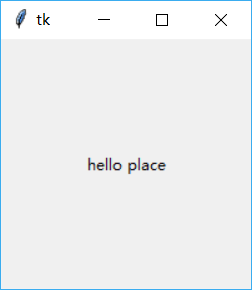relx,rely 指定组件放置的绝对位置,范围为(0-1.0)

使用 place 同时指定多个组件

代码:

```import tkinter as tk
root = tk.Tk()
root.geometry('500x80')  # 设置初始root大小
v = tk.IntVar()  # 引用变量数字
lb = tk.Label(root, text='hello place')
# 使用相对坐标(0.5,0.5)将 Label 放置到(0.5*sx,0.5.sy)位置上
for i in range(5):
variable=v,
value=i,  # 设置变量值为i
).place(x=80*i, anchor='nw')
root.mainloop()```

结果:同时使用相对和绝对坐标

同时设置 relx,rely 和 x,y 的值

代码:

```import tkinter as tk
root = tk.Tk()
root.geometry('500x200')  # 设置初始root大小
v = tk.IntVar()  # 引用变量数字
lb1 = tk.Label(root, text='hello place',fg='red')
lb2 = tk.Label(root, text='hello place',fg='blue')
# 先设置相对坐标为(0.5,0.5),再使用(5,10)将坐标作偏移(5,10)
lb1.place(relx=0.5,rely=0.5,anchor='center',x=5,y=10)

# 先设置相对坐标为(0.5,0.5),再使用(25,30)将坐标作偏移(25,30)
lb2.place(relx=0.5,rely=0.5,anchor='center',x=25,y=30)
root.mainloop()```

结果: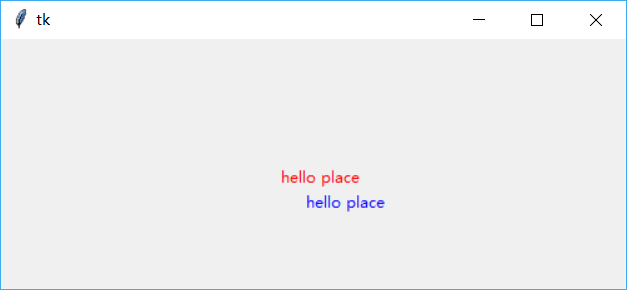同时使用相对和绝对坐标时，相对坐标优先操作，然后是在这个相对坐标的基础上进行偏移

使用 in 来指定放置的容器

使用 in 属性来指定放置到的容器是那一个

代码:

```import tkinter as tk
root = tk.Tk()
root.geometry('500x200')  # 设置初始root大小
v = tk.IntVar()  # 引用变量数字
lb1 = tk.Label(root, text='hello place',fg='red')
bt1 = tk.Button(root, text='hello button',fg='blue')
# 创建一个 Label
lb1.place(relx=0.5,rely=0.5,anchor='center')

# 在 root 同创建一个 Button，目的是与 bt1相比较
bt2 = tk.Button(root, text='button in root',fg='yellow')
bt2.place(anchor='w')
# 在 Label 中创建一个 Button
bt1.place(in_=lb1, anchor='w')
root.mainloop()```

结果: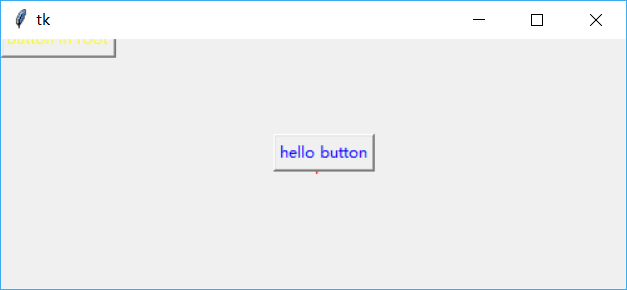注意 bt2放置的位置是在 root 的(0,0)处，而 button1放置的位置是在 lb1的(0,0)处，原因是由于 bt1使用了 in 来指定放置的窗口为 lb1

深入 in 用法

使用 in 属性来指定放置到的容器是那一个，仅能是其 master

代码:

```import tkinter as tk
root = tk.Tk()

# 创建两个 Frame 用作容器
fm1 = tk.Frame(root,bg='red',width=40,height=40)
fm2 = tk.Frame(root,bg='blue',width=40,height=40)

# 再在 fm1中创建一个 fm3
fm3 = tk.Frame(root,bg='yellow',width=20,height=20)

# 创建一个 Label,它的 master 为 fm1
lb1 = tk.Label(fm1,text='hello place',fg='green')
lb1.place(in_=fm1, relx=0.5,rely=0.5,anchor='center')

# 创建一个 Button，它的 master 为 fm1
bt1 = tk.Button(fm1, text='hello place', fg='red')

# 将 bt1放置到 fm2中，程序报错
# 去掉下面这条语句就可以使用了，可以看到 lb1已经正确的放置到 fm1的中心位置了
# bt1.place(in_=fm2, anchor='w')

# 将 bt1放置到 fm3中，程序报错
# bt1.place(in_=fm3, anchor='w')

fm1.pack()
fm2.pack()
fm3.pack()
root.mainloop()```

结果: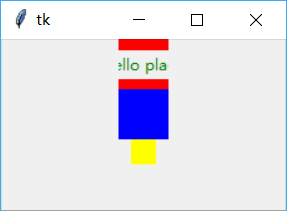in 不是可以随意指定放置的组件的，如果使用 in 这个参数这个组件必需满足：是其父容器或父容器的子组件

事件与 Place 结合使用

最后使用两个 place 方法来动态改变两个 Frame 的大小

代码:

```import tkinter as tk
root = tk.Tk()
split=0.5  # 定义变量

# 创建两个 Frame 用作容器
fm1 = tk.Frame(root,bg='red',width=40,height=40)
fm2 = tk.Frame(root,bg='blue',width=40,height=40)

# 单击 fm1时增大它的占有区域0.1
def incFm1(event):
global split
if split < 1:
split += 0.1
fm1.place(rely=0,relheight=split,relwidth=1)
fm2.place(rely=split, relheight=1-split, relwidth=1)

# 单击 fm2时增大它的占有区域0.1
def incFm2(event):
global split
if split > 0:
split -= 0.1
fm1.place(rely=0, relheight=split, relwidth=1)
fm2.place(rely=split, relheight=1-split, relwidth=1)

# 这两语句要使用，不然开始看不到两个 frame，也就没法点击它们了
fm1.place(rely=0, relheight=split, relwidth=1)
fm2.place(rely=split, relheight=1-split, relwidth=1)

# 绑定单击事件
fm1.bind('',incFm1)
fm2.bind('',incFm2)

root.mainloop()```

结果: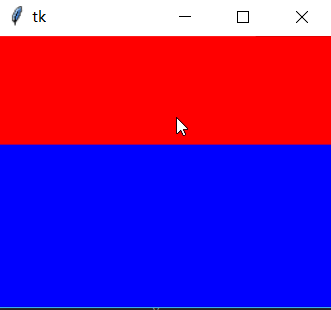为 SplitWindow 的原型了，再改动一下就可以实现一个 SplitWindow 了